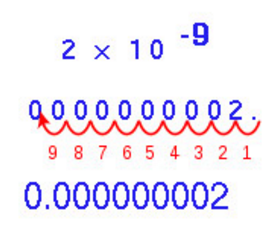# Standard Form Formula

Sometimes we come across a very large number, which needs to be written in a form much smaller and understandable by the universe. When a large number is reduced in its decimal notation accompanied by an exponential, it is called standard form. Sometimes standard form is also known as exponential notation or scientific notation.

• Step one: Put a decimal point right after the first digit of a number from its right
• Step two: Count the number of places after the decimal, that will be the scientific notation.
• Step three: Drop the zeroes if any present after the decimal at the end to the end of the number

Example: The standard form of 1220 can be written as

$$\begin{array}{l}1.22 \times 10^{3}\end{array}$$

To understand negative numbers standard form, refer the image below:To accommodate the digits after decimal places we use this method.

### Solved Examples

Question: Write 4253 in standard form?

Solution:

$$\begin{array}{l}4.253 \times 1000\end{array}$$
$$\begin{array}{l}4.253 \times 10^{3}\end{array}$$